# Workshop Chapter 11. Using Math to Create a Circular Slider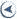CONTENTS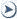## Workshop Chapter 11. Using Math to Create a Circular Slider

By now, you're probably quite familiar with calculating percentages based on minimum and maximums. You're also probably quite comfortable writing scripts for the enterFrame and mouseMove events. This workshop chapter involves using just a few trigonometry concepts to calculate angles and to specify locations around a circular path. Suppose that you have two known points, such as the center of a clip and the current position of the mouse. Calculating the angle of a line drawn through those points is the main task in this workshop. Although the applications of such a trick can include creating eyeballs that appear to follow the mouse movements (see Figure W11.1), we can actually use this knowledge for something more practical: a slider that moves along a curved path.

##### Figure W11.1. Making eyeballs follow the mouse is one of the fun things you can do with math.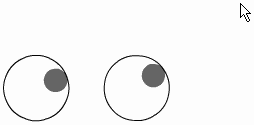It turns out that the actual math involved in this workshop chapter isn't that bad. However, if you want, you can brush up on some of the Math object methods especially Math.atan2(), the first trigonometry function I learned to love by reviewing Chapter 5, "Programming Structures."

Here are the steps for creating a curved slider:

1. First, to get a sense of the formula we'll be using, create an eyeball shape: Draw a small dark circle inside a larger light circle, and make the dark circle touch the inside edge of the right side of the light circle (see Figure W11.2).

##### Figure W11.2. Position the small dark circle inside the right edge of the larger circle.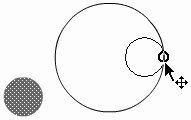2. Select everything and convert it to a Movie Clip called "Eyeball." Attach the following code to the Movie Clip instance:

`onClipEvent (mouseMove) {   var radians = Math.atan2(_root._ymouse-this._y, _root._xmouse-this._x);    var degrees=radians/(Math.PI/180);    this._rotation=degrees;    updateAfterEvent();}`

Every time the mouse moves, this code will first assign the variable radians to the result of the Math.atan2() method. The two parameters provided to Math.atan2() are the x and y difference between where the mouse is and the center of the Eyeball clip. (For example, the y difference is _root._ymouse - this._y.) As you recall from Chapter 5, the Math.atan2() function returns an angle based on the length of the sides of a right triangle, even if those lengths are negative. Realize that for any angle you can draw vertical and horizontal lines to create a right triangle. Those two sides are provided for by the parameters in the Math.atan2() function. Also remember from Chapter 5 that the angle returned is in the form of radians.

The second line of this script uses a standard formula to convert radians to degrees. Those degrees are used to set the angle of the Eyeball clip.

It's kind of cool: You can copy as many instances of the Eyeball clip as you want, and they'll all calculate the correct _rotation to follow the mouse pointer.

So we can make eyeballs, so what? For our slider, this bit of math will come in handy. While the user drags, we can calculate the angle from any known point such as the center of the circle around which the user is dragging to the mouse (see Figure W11.3).

##### Figure W11.3. We're going to make a draggable slider that follows the radius of a circle.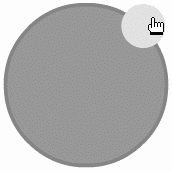With that angle in hand, we can calculate where the slider should be positioned. We want the user to be able to grab a small circle (think of the moon); as the user drags that circle, we position it in the correct place along a larger circle (think of the moon's orbit).

Actually, we could solve this exercise just by placing the moon off center within a clip (similar to the way the pupil was off center within the Eyeball symbol). However, this seemingly easier approach would require that any adjustments to the radius be made by editing the contents of our moon symbol. Besides, we've already learned how to rotate an off-center symbol (the Eyeball). Positioning a clip on a circular path is entirely different.

3. In the main timeline, draw a large circle and convert it to a Movie Clip. Give it an instance name of theCircle. It is the perimeter of this instance that we'll use for the draggable clip's path.

4. Copy and paste the instance of theCircle, scale it down, tint it, and then change its instance name to slider. You can also snap its center to a point on the edge of the theCircle instance.

5. Now go to the frame script in the main timeline (where we'll put all the remaining code for this exercise) and type the following script to initialize some variables:

`centerX=theCircle._x;  centerY=theCircle._y;  radius = theCircle._width/2;`

To calculate the angle (between the center of the theCircle clip and the mouse), we need to know the center of the circle. Also, to position the slider instance in the correct location, we need to know the radius of the circle (that is, half its width). These variables just save a bit of typing later.

6. Before we actually start calculating angles and positioning the slider instance, let's get the triggers in place. All we'll do is assign an onMouseMove callback to the slider instance when the user presses on it. In addition, we'll clear this callback when the user releases the mouse. Type the following code below what you already have:

`slider.onPress=function(){slider.onMouseMove=dragTime}  slider.onRelease=function(){slider.onMouseMove=null}  slider.onReleaseOutside=function(){slider.onMouseMove=null}`

The function dragTime will be defined in a second. Naturally, in place of the word dragTime we could have defined the function right there but for readability we'll do it next. Quite simply, this code says that while the user is dragging (that is, after an onPress event), the dragTime function will be tied to every mouseMove. Then, when the user lets go of the mouse, there will be no onMouseMove callback.

7. The dragTime() function will have one primary objective: to position the slider instance. It will take a couple of steps, of course, so first write the following skeleton into which we'll add one line at a time:

`function dragTime(){ }`
8. We can use the atan2() method to determine the angle between the center of the circle and the mouse pointer. Here, we'll store the angle in a variable called radians (which goes inside the dragTime() function):

`var radians = Math.atan2(_root._ymouse-centerY, _root._xmouse-centerX);`

Note that this is the same code that we used in the Eyeball example.

9. With the angle stored in the radians variable, we can now position the slider. Interestingly, because we're not rotating anything (that is, setting its _rotation property), we may never need to convert to degrees. Actually, doing so prematurely would mess us up. Check out these two lines that go right below the radians assignment:

`this._y = centerY+Math.sin(radians)*radius;  this._x = centerX+Math.cos(radians)*radius;`

First look at the code to the right of the plus sign. When provided with an angle (in radians), the Math.sin() method returns the vertical proportion. Remember that sine returns the "opposite/hypotenuse." So, if you imagine a triangle that varies from 0 degrees to 90 (see Figure W11.4), you should see that "opposite/hypotenuse" is 1 at 90 degrees (because the opposite and hypotenuse are equal). At 0 degrees, sine returns 0 because the opposite is 0 height. Anyway, all variations between 0 and 1 are some percentage. The reason we multiply the sine (in line 1) by radius is because at 90 we want the _y to be the full radius (vertically). When at 0 degrees, we want the _y to be zero. The entire first line says, "set the _y to the center of the circle plus the appropriate proportion of the radius based on the sine." If that doesn't make sense, you can read more about this topic in Chapter 5. Also notice that we don't bother converting to degrees. The Math.atan2() method returns radians, and both Math.cos() and Math.sin() accept radians, so there's no need to bother with the "degrees" middleman, if you will.

##### Figure W11.4. Visualizing trigonometry functions helps deduce the expressions you write.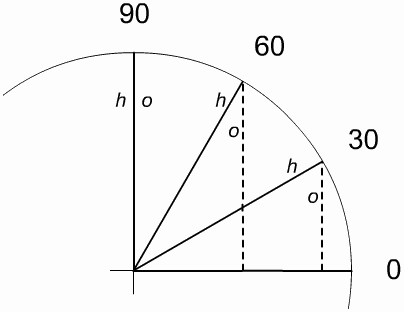10. Throw in an updateAfterEvent() below the three lines you've added to the dragTime() function.

We're done early, so let's add a feature that will calculate what percentage the way around the circle the slider is currently positioned. Perhaps you want to make this control the volume or some other effect.

11. To display the percentage, place a Dynamic Text field on the Stage and give it an instance name of percent_txt.

12. Because I know the forthcoming discussion will be easier for you if we go back to degrees, add the following lines of code inside the dragTime() function:

`var degrees = radians/(Math.PI/180);  _root.percent_txt.text=degrees;`

After converting to degrees, we set the text in the percent_txt field to display the degrees as we drag. Notice that when you test the movie, dragging clockwise from nine o'clock increases degrees from 0 to 180, whereas moving counterclockwise reduces a negative number from 0 to 180. I suppose it makes sense. My idea was to display 0 100. I'd expect to at least see 0 360, but no dice. We'll need to adjust the display.

13. We can make the display of degrees range from 0 to 360 by inserting an if statement between the two statements you inserted in Step 12:

`var degrees=radians/(Math.PI/180);  if (degrees<0){   degrees=360+degrees;  }  _root.percent=degrees;`

This code simply says that if degrees is less than 0, set degrees to 360 plus degrees (which is negative). That is, 180 (three o'clock) turns to 180; 90 (six o'clock) changes to 270; and so on (see Figure W11.5).

##### Figure W11.5. The original degree values on the outside of the circle, including negative numbers, can be converted to all positive numbers as shown on the inside of the circle by using a simple formula.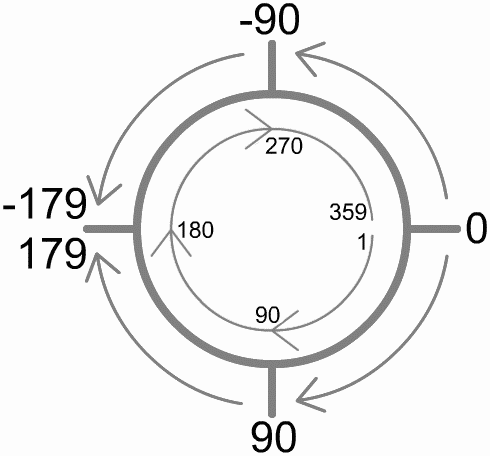14. To make the percent variable show a number between 0 and 99, we can change the last line of the script to read as follows:

`_root.percent_txt.text=Math.floor(100*degrees/360);`

This statement says that 360 divided into degrees times 100 gives us a percentage. You can add 1 outside the Math.floor() method if you want to display values from 1 through 100.

You can just as easily use these calculations to set any property of any other clip. For example, if you have a clip named it, you can add this line of code:

`_root.it._alpha= Math.floor(100*degrees/360);`
15. If you don't like the fact that the scale starts at three o'clock, you can use the following technique to adjust the degrees found by any amount you want. Suppose that we want to add 90 degrees from every value to make twelve o'clock become the zero point. After all, before we converted to an actual percentage, twelve o'clock was displaying 90 (refer to Figure W11.5). To accomplish this task, add what you want (90 in this case), and our current if statement will adjust for any negative numbers. Place the following code right before the line starting if (degrees<0)...:

`degrees+=90;`

All this does is add 90 from degrees so that twelve o'clock is the zero point. As long as you do this before that if statement, you can subtract any value from the degrees calculated.

This discussion was a little easier for you to read and for me to write after we converted to degrees. However, unless you are setting _rotation, which is the one part of Flash that requires numbers in degrees, you probably will never need to convert to degrees. If you remember that there are 2*Math.PI radians in a circle, you can perform any of the code in steps 12 15. For example, here is the same exact code without ever translating radians to degrees:

`radians+=(Math.PI/2);  if (radians<0){   radians=(2*Math.PI)+radians;  }  _root.percent_txt.text=Math.floor(100*radians/(2*Math.PI));`

Notice that this code has one less line than the original version because we're not translating to degrees. The first line effectively adds 90 degrees from the radians found because Math.PI/2 is equivalent to 90 degrees. If radians is less than 0, we subtract radians from 2*Math.PI in the same way we subtracted degrees from 360 earlier. Finally, to figure out the percentage, we divide radians by 2*Math.PI instead of dividing degrees by 360.

With the exception of a little bit of math, this workshop turned out to be pretty easy. We'll use some of the same knowledge in Workshop Chapter 12, "Developing Time-Based Animations," to rotate a clip and make it follow a path. The main goal of that workshop chapter, however, is to make sure that the clip rotates at a perfect rate, regardless of frame rate or the computer's performance. You will get to use some of the same math, though.CONTENTSActionScripting in MacromediaR FlashT MX
ISBN: N/A
EAN: N/A
Year: 2001
Pages: 41